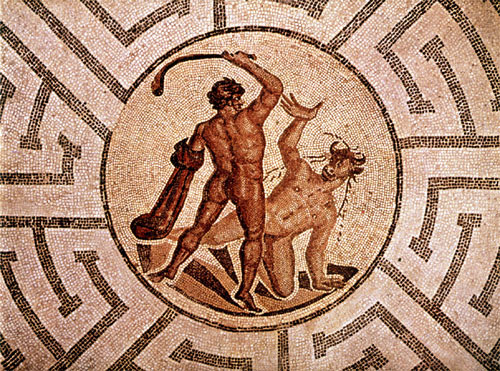# Can Theseus Hide From The Minotaur?

Logic Level 3Assume a labyrinth layout in the form of chambers. In a certain labyrinth map, there are $m\times m, (m\geq2)$ , chambers in the form of a large square. Now, the chambers were labelled as $1,2,3,\ldots, m^{2}$, row by row, from left to right. Now, say the Minotaur starts in chamber $1$. There is a set of rules for the Minotaur to move through chambers, considering that all the chambers are interconnected.

• If the Minotaur is in in chamber $n, 1\leq n \leq m^{2}$ and $n\equiv 0 \pmod{4}$, then move to the chamber in the row above ($\uparrow$).
• If the Minotaur is in in chamber $n, 1\leq n \leq m^{2}$ and $n\equiv 1 \pmod{4}$, then move to the chamber on the right ($\rightarrow$).
• If the Minotaur is in in chamber $n, 1\leq n \leq m^{2}$ and $n\equiv 2 \pmod{4}$, then move to the chamber in the row below ($\downarrow$).
• If the Minotaur is in in chamber $n, 1\leq n \leq m^{2}$ and $n\equiv 3 \pmod{4}$, then move to the chamber on the left ($\leftarrow$).
• If the directions lead the Minotaur out of the labyrinth, then the Minotaur will scroll to the chamber on the opposite end of the row or column, depending on the direction in which the Minotaur leaves the labyrinth.
• There is no limit to how many times the Minotaur will move.

Say that Theseus is forced to stay in one chamber of the labyrinth. There is a chamber numbered $a$, such that no matter what value $m$ is (i.e. the dimensions of the square), the Minotaur will never enter this chamber. Find the value $a$.

I was doodling in my notebook, and realized something interesting.

×